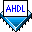## Creating Counters

 See AlsoCounters use sequential logic to count clock pulses. Some counters can count forward and backward, and can be loaded with data and cleared to zero. Counters can be defined with D flipflops (`DFF`, `DFFE`, and `DFFEA` ) and If Then Statements or with the `lpm_counter` function.

The ahdlcnt.tdf file shown below implements a 16-bit loadable up counter that can be cleared to zero.

```SUBDESIGN ahdlcnt
(
clk, load, ena, clr, d[15..0] : INPUT;
q[15..0]                      : OUTPUT;
)

VARIABLE
count[15..0]                  : DFF;

BEGIN
count[].clk = clk;
count[].clrn = !clr;

count[].d = d[];
ELSIF ena THEN
count[].d = count[].q + 1;
ELSE
count[].d = count[].q;
END IF;

q[] = count[];
END;
```

In this file, 16 D flipflops are declared in the Variable Section and assigned the names `count0` through `count15`. The If Then Statement determines the value that is loaded into the flipflops on the rising clock edge (such as, if `load` is driven by `VCC`, the flipflops are assigned the value of `d[]`).

The lpm_cnt.tdf file shown below uses the `lpm_counter` function to implement the same functionality as ahdlcnt.tdf:

```INCLUDE "lpm_counter.inc";
SUBDESIGN lpm_cnt
(
clk, load, ena, clr, d[15..0] : INPUT;
q[15..0]                      : OUTPUT;
)
VARIABLE
my_cntr: lpm_counter WITH (LPM_WIDTH=16);

BEGIN
my_cntr.clock  = clk;